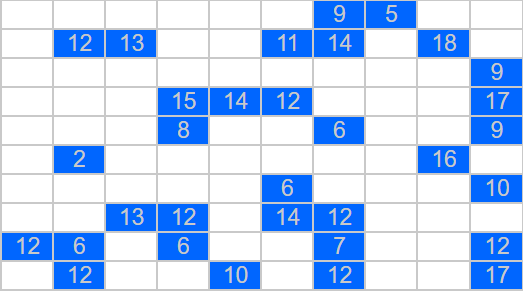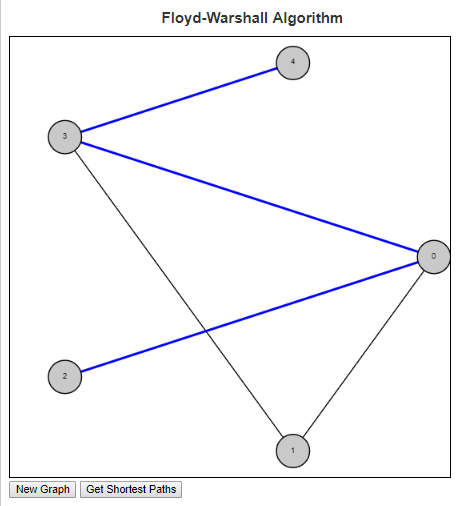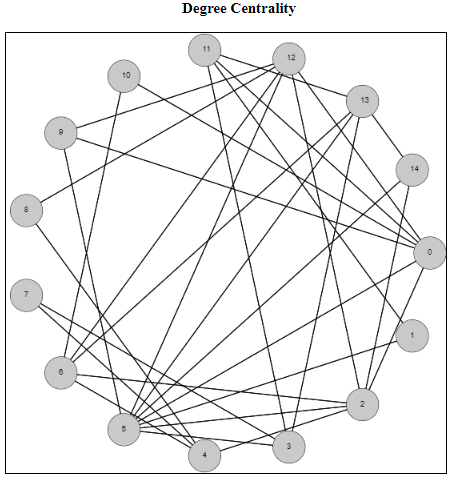# Pascal’s Triangle

I had a recent conversation with a friend who asked me “what makes number theory interesting?”. I loved the question, mainly because it gave me an opportunity to talk about math in a positive manner. More importantly though, it was an opportunity to talk about one of my favorite courses in mathematics (along with discrete mathematics and set theory). As much as the current day seems to focus on joining Number Theory with Cryptography, when I answered this question I wanted to make sure I didn’t go that route. Numbers are beautiful in their own right, and one of the things about Number Theory that was so interesting was simply the ability to look at all the different questions and patterns and properties of numbers discovered.

To answer this question, I started listing numbers to see if she noticed a pattern, but I did it with a “picture”.

.
..

….
…..
……

• How many dots will go on the next line
and
• After each line how many dots have been drawn in total?

Dots# on this line# in total
.11
..23
36
….410
…..515

There were a lot of directions I could have taken this conversation next, but I decided to stay in the realm of triangles and discuss Pascal’s triangle. This is a triangle that begins with a 1 on the first row and each number on the rows beneath is the sum of the two cells above it, assuming that cells not present have a value of zero.

So the first five rows of this triangle are

1
11
121
1331
14641

This is an interesting and beautiful triangle because of just the number of patterns you can see in it.

• Obviously there are ones on the outside cells of the triangle.
• One layer in, we get what are called the Natural or Counting numbers (1, 2, 3, 4, 5, …) .
• One layer in, we can start to see the list of numbers that I was showing my friend (1, 3, 6, 10, 15, …).

There are several other properties of this triangle and I wanted to allow users to begin to see them, so I wrote a script highlighting some of these patterns.

# Set Partition Problems

This is my first post of 2019 and my first post in a while. There was one posted a few months ago, but not really geared towards the algorithms and learning focus of the site. I have been doing a lot of coding in my spare time, but honestly life has just gotten in the way. Its not a bad thing, but life is life and sometimes I have to prioritize the things. In particular, I have been having ideas and actually coding things up but the time it takes to clean up code, write a blog entry and finding a nice way to visualize these things has been something that I haven’t been able to really focus on as much as I’ve wanted to.

That said, I want to talk to you about the Set Partition Problem today. You can go to Wikipedia to get more information about this problem, but I will give you a brief introduction to it and then talk about two different approaches to it. The problem assumes that we are given as input a (multi)-set S. The reason we say it is a multi-set and not a simple set is because we can have the same element appear multiple times in the set. So if S1 = {2} and S2 = {2, 2}, then although as sets they are both equal to the set {2} = S1, as multi-sets allow for multiple instances of an element. The elements of S are assumed to be positive integers.

So given this multi-set S, we ask the question of can the elements of S be divided into two smaller multi-sets, C1 and C2 where

• C1 [union] C2 = S
• C1 [intersect] C2 = [empty set]
• [Sigma]_[x in C1] = [Sigma]_[x in C2].C1 [union] C2 = S, C1 [intersect] C2 = [empty set], and [Sigma]_[x in C1] = [Sigma]_[x in C2]

The first two bullets above say that the sets C1 and C2 form a partition of S. The third bullet says that the sums of the elements in the two children multi-sets are equal.

This problem is known to be NP Complete. This means that it is one of the more difficult decision problems. Because of this finding an algorithm that solves this problem exactly will generally take a long running time. And finding an algorithm that runs quickly will more than likely be incorrect in some instances.

I will show you two approaches to this problem. One is based on Dynamic Programming (DP) and one is based on Greedy Algorithms. The DP version solves the problem exactly but has a slow running time. The greedy algorithm is fast but is not guaranteed to always give the correct answer.

Dynamic Programming is based on the principle of optimality. This says that in order to have a correct solution to the overall problem, we need optimal solutions to all subproblems of this problem. This is done by keeping track of a table which can be used for looking up these subproblems and the optimal solutions to these subproblems.

For this problem, we will build a table where the rows represent the final sums and columns represent subsets of the given multi-set (containing the first 0…n elements). The question we are repeatedly asking is “can we find a subset of the set in this column whose sum is exactly the given rowsum?” Here is an example of the DP algorithm on the multi-set {5, 6, 5, 6, 7}.

Greedy Algorithms are generally based on sorting the elements based on some principle and using that to try to answer the underlying question. The main problem with this approach is that it is very short sided because they do not look at the overall picture. Such algorithms are known for finding local optima that are not always globally optimal. The benefit to these problems though is that they are generally easier to code and easier to understand.

For the Set Partition Problem , the Greedy approach is to sort elements in descending order. Once this is done, the goal is to keep two subsets while iterating through the array, adding the element to the smaller of the two sets whenever possible.

For more examples, check out Set Partition Problems

# Range Puzzles

Range PuzzlesI have always liked puzzles. I really enjoy discovering a new puzzle. Sometimes when I have discovered a new puzzle, I enjoy it so much that I can’t seem to do enough of them. In such cases, I quickly run out of these puzzles to do and find myself looking for either a new puzzle or a way to generate them on my own.

Such was the case when I discovered the “Range Puzzles”. The rules are simple:

Every cell is marked either blue or gray
No two gray cells can be next to one another
The grid must be a connected (i.e. there is always a path from every cell to every other cell using horizontal and vertical connections.
Some cells have a number inside. This indicates the number of cells that can be viewed (horizontally and vertically in both directions) by this cell, including the cell itself.

To try out these puzzles, visit Range Puzzles at LEARNINGlover.com

# Floyd-Warshall Shortest PathsThe Floyd Warshall algorithm is an all pairs shortest paths algorithm. This can be contrasted with algorithms like Dijkstra’s which give the shortest paths from a single node to all other nodes in the graph.

Floyd Warshall’s algorithm works by considering first the edge set of the graph. This is the set of all paths of the graph through one edge. Node pairs that are connected to one another through an edge will have their shortest path set to the length of that edge, while all other node pairs will have their shortest path set to infinity. The program then runs through every triplet of nodes (i, j, k) and checks if the path from i to k and the path from k to j is shorter than the current path from i to j. If so, then the distance and the path is updated.

So lets consider an example on the graph in the image above. The edge set of this graph is E = {(0, 1), (0, 2), (0, 3), (1, 3), (3, 4)}. So our initial table is:

 0 1 2 3 4 0 inf (0, 1) (0, 2) (0, 3) inf 1 (0, 1) inf inf (1, 3) inf 2 (0, 2) inf inf inf inf 3 (0, 3) (1, 3) inf inf (3, 4) 4 inf inf inf (3, 4) inf

As we look to update the paths, we first look for routes that go through node 0:

Because node 0 connects to both node 1 and node 2, but node 1 does not connect to node 2, we have the following truth holding in the matrix above:
cost(0, 1) + cost(0, 2) < cost(1, 2), so we can update the shortest path from node 1 to node 2 to be (1, 0, 2).

Because node 0 connects to both node 2 and node 3, but node 2 does not connect to node 3, we have the following truth holding in the matrix above:
cost(0, 2) + cost(0, 3) < cost(2, 3), so we can update the shortest path from node 2 to node 3 to be (2, 0, 3).

Because node 3 connects to both node 0 and node 4, but node 0 does not connect to node 4, we have the following truth holding in the matrix above:
cost(0, 3) + cost(3, 4) < cost(0, 4), so we can update the shortest path from node 0 to node 4 to be (0, 3, 4).

Because node 3 connects to both node 1 and node 4, but node 1 does not connect to node 4, we have the following truth holding in the matrix above:
cost(1, 3) + cost(3, 4) < cost(1, 4), so we can update the shortest path from node 1 to node 4 to be (1, 3, 4).

Because node 3 connects to both node 2 and node 4, but node 2 does not connect to node 4, we have the following truth now holding:
cost(2, 3) + cost(3, 4) < cost(2, 4), so we can update the shortest path from node 2 to node 4 to be (2, 0, 3, 4).

The final table giving the list of shortest paths from every node to every other node is given below.

 0 1 2 3 4 0 inf (0, 1) (0, 2) (0, 3) (0, 3, 4) 1 (0, 1) inf (1, 0, 2) (1, 3) (1, 3, 4) 2 (0, 2) (1, 0, 2) inf (2, 0, 3) (2, 0, 3, 4) 3 (0, 3) (1, 3) (2, 0, 3) inf (3, 4) 4 (0, 3, 4) (1, 3, 4) (2, 0, 3, 4) (3, 4) inf

To see more examples and to help answer questions, check out the script in my examples section on the Floyd-Warshall algorithm

# Degree Centrality of a GraphI wanted to spend some time on centrality measures of a graph. These are measurements of how important each node (or edge) is to the overall graph. But how do we define, or determine, importance? There is no unique way to answer this question, so there are varying metrics for measuring centrality. Which one you choose depends on several factors including how many other nodes of the graph are included, as well as the run time of the metrics you’re considering.

I have just published a script focusing on the degree centrality metric. The degree centrality metric is called a “walk metric” because it determines how important a node is by how many other nodes that can be reached by walks of up to a certain length. Lets look at the definition of the degree of a node to see if we can understand why it is called a walk metric.

In an undirected graph G = (V, E), the degree of a node u [in] V is the |{v | (u, v) [in] E}|. This is the size of the set of nodes that are connected to node u via a single edge. Another way of describing a single edge is a walk of length one. So the degree metric measures the importance of a node by the number of unique walks of length one.

The normalized degree centrality of a node v in a graph G = (V,E) measures how many nodes are connected to the node v, compared to the maximum possible number of edges that can be connected to this node. Because we are dealing with simple undirected graphs (at most a single edge between any two distinct vertices), this maximum possible number will always be |V – 1|. So the normalized degree can be calculated by dividing the degree of the node (the number of nodes it is connected to) by |V – 1|.

So for the example above, the node 0 has degree 6 because it is connected to nodes 2, 5, 9, 10, 11, and 12. There are 15 total nodes in this graph, so to calculate the normalized degree centrality of the node 0, it will be 6 / 14, which rounds to 0.428571.

To see more examples and to help answer questions, check out the script in my examples section on degree centrality